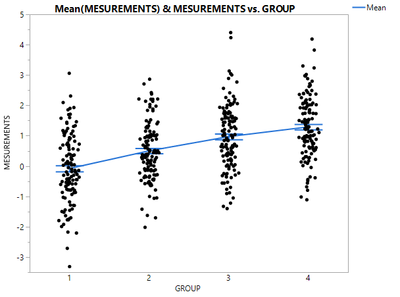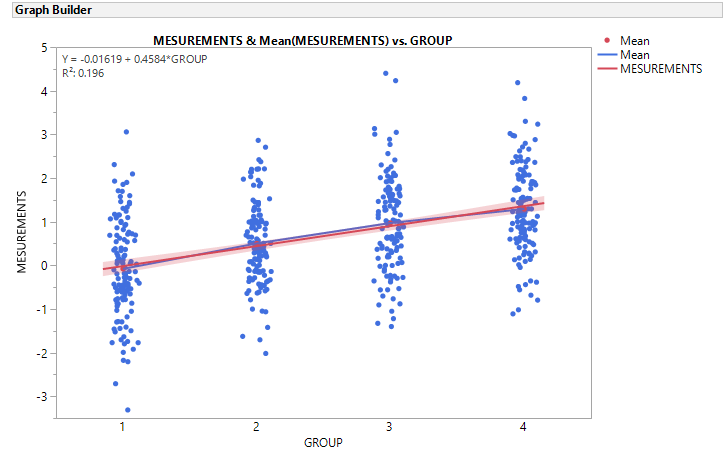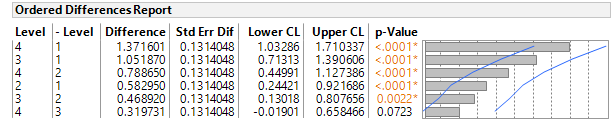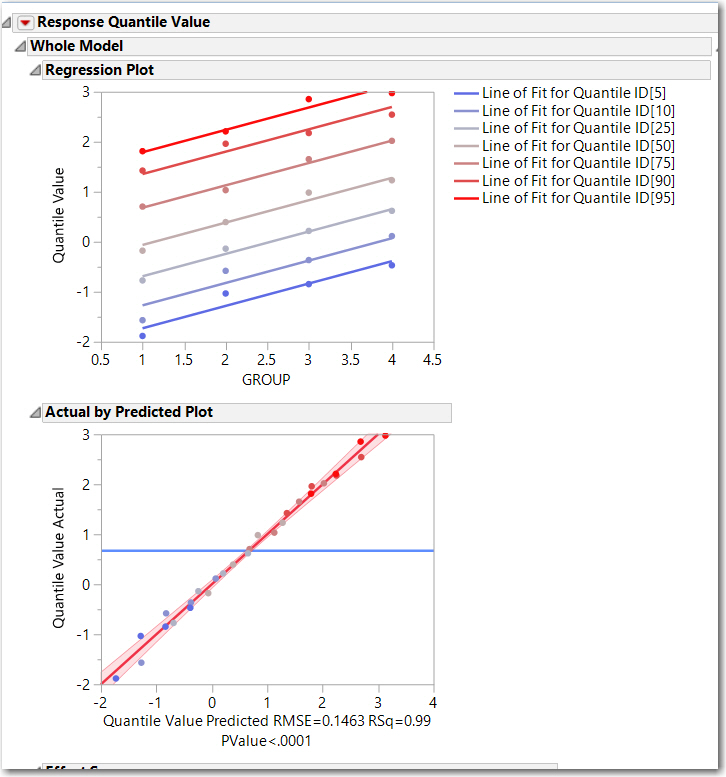It’s World Statistics Day! To honor the theme of the day, the JMP User Community is having conversations about the importance of trust in statistics and data. And we want to hear from you! Tell us the steps you take to ensure that your data is trustworthy.
Choose Language Hide Translation Bar
Highlighted

## Trend Evaluation where X = Ordinal and Y = Continuous?

Hi JMP Community,

I would like to know if it is possible to evaluate(in JMP 13.1) trends in a data where X is ordinal (4 Groups) and Y is continuous (see attached file).Based on a visual inspection, it appears that the means of Measurements are progressively higher with each group but I have not been able to find a method to evaluate this formally.

TS

Thierry R. Sornasse
1 ACCEPTED SOLUTION

Accepted Solutions
Highlighted

## Re: Trend Evaluation where X = Ordinal and Y = Continuous?

@Thierry_S, in JMP 13 Graph Builder, you can add a Line of Fit via  the UI or use this saved script

``````Graph Builder(
Variables( X( :GROUP ), Y( :MESUREMENTS ) ),
Elements(
Points( X, Y, Legend( 3 ) ),
Points( X, Y, Legend( 4 ), Summary Statistic( "Mean" ) ),
Line( X, Y, Legend( 5 ) ),
Line Of Fit( X, Y, Legend( 6 ), R²( 1 ), Equation( 1 ) )
),
SendToReport( Dispatch( {"Line Of Fit"}, "", OutlineBox, {Close( 0 )} ) )
)``````

Here is the result.However, I would follow this approach:

• Use Analyze > Fit Y by X to run a one way ANOVA, the means should increase with Group and you should test for global significance difference in the means then compare the groups.  For example, does Group 3 have a statistically different mean that Group 4, etc.
• Look at the successive differences in the group means ( 2-1, 3-2, 4-3), they are are all positive (0.58, 0.47, 0.32). 4-3 is not significant at 0.05, however, the p-value is 0.07 which provides some indication of a trend, but maybe slowing.• I would not recommend a higher order model without more context on this ordinal Group. For example, this could be the luck of the draw, that the order of these four groups result is 1,2,3,4  one out of 24  possible ordering (4! different outcomes).  If there is a "cause" for this ordering like dose, or repetition (learning) or some social group, the model should be built using the "cause" variables.
• A simple method other than Graph Builder  to support  the "trend" is to right click on the means and standard deviation table and select Make into Data Table. Then use Fit Y by X with the Mean as Y and Group as X (make it continuous) the ft the line

However, when looking for a shift in the "distribution" of these groups, I like to take a different approach:

• From your table, compute the distribution quantiles for each Group, 95, 90, 75, 50, 25, 10, 5 using Tables > Summary. The resulting table needs a little work: stack the quantiles, simplify the Quantile ID.
• Then I like to look at the relationship of the 90th percentile (for example) across the groups. Is it changing as much of as the mean?
• The summary QuantizedTable and the script to create the analysis below is attached to the table. This graphic indicates that the entire distribution is shifting, not just the mean... which lends strength to the argument of a trend by group

Let me reiterate, this is an exploratory analysis. Any modeling or further investigation should be placed on the drivers/cause of the shift.Highlighted

## Re: Trend Evaluation where X = Ordinal and Y = Continuous?

@Thierry_S, in JMP 13 Graph Builder, you can add a Line of Fit via  the UI or use this saved script

``````Graph Builder(
Variables( X( :GROUP ), Y( :MESUREMENTS ) ),
Elements(
Points( X, Y, Legend( 3 ) ),
Points( X, Y, Legend( 4 ), Summary Statistic( "Mean" ) ),
Line( X, Y, Legend( 5 ) ),
Line Of Fit( X, Y, Legend( 6 ), R²( 1 ), Equation( 1 ) )
),
SendToReport( Dispatch( {"Line Of Fit"}, "", OutlineBox, {Close( 0 )} ) )
)``````

Here is the result.However, I would follow this approach:

• Use Analyze > Fit Y by X to run a one way ANOVA, the means should increase with Group and you should test for global significance difference in the means then compare the groups.  For example, does Group 3 have a statistically different mean that Group 4, etc.
• Look at the successive differences in the group means ( 2-1, 3-2, 4-3), they are are all positive (0.58, 0.47, 0.32). 4-3 is not significant at 0.05, however, the p-value is 0.07 which provides some indication of a trend, but maybe slowing.• I would not recommend a higher order model without more context on this ordinal Group. For example, this could be the luck of the draw, that the order of these four groups result is 1,2,3,4  one out of 24  possible ordering (4! different outcomes).  If there is a "cause" for this ordering like dose, or repetition (learning) or some social group, the model should be built using the "cause" variables.
• A simple method other than Graph Builder  to support  the "trend" is to right click on the means and standard deviation table and select Make into Data Table. Then use Fit Y by X with the Mean as Y and Group as X (make it continuous) the ft the line

However, when looking for a shift in the "distribution" of these groups, I like to take a different approach:

• From your table, compute the distribution quantiles for each Group, 95, 90, 75, 50, 25, 10, 5 using Tables > Summary. The resulting table needs a little work: stack the quantiles, simplify the Quantile ID.
• Then I like to look at the relationship of the 90th percentile (for example) across the groups. Is it changing as much of as the mean?
• The summary QuantizedTable and the script to create the analysis below is attached to the table. This graphic indicates that the entire distribution is shifting, not just the mean... which lends strength to the argument of a trend by group

Let me reiterate, this is an exploratory analysis. Any modeling or further investigation should be placed on the drivers/cause of the shift.Article Labels

There are no labels assigned to this post.